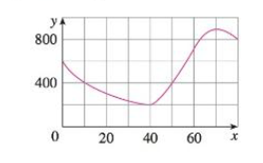Chapter 2.7, Problem 18E### Single Variable Calculus: Early Tr...

8th Edition
James Stewart
ISBN: 9781305270343

#### Solutions

Chapter
Section### Single Variable Calculus: Early Tr...

8th Edition
James Stewart
ISBN: 9781305270343
Textbook Problem

# The graph of a function f is shown.(a) Find the average rate of change of f on the interval [20, 60].(b) Identify an interval on which the average rate of change of f is 0.(c) Which interval gives a larger average rate of change, [40, 60] or [40, 70]?(d) Compute f ( 40 ) − f ( 10 ) 40 − 10 ; what does this value represent geometrically?(a)

To determine

To find: The average rate of change of f on the interval [20,60].

Explanation

Given:

The graph of the function y=f(x).

From the given graph, it is observed that the domain of the function is on the interval [0,80] and the co-domain of the function is [0,1000].

Formula used:

The average rate of change of y with respect to x on the interval [x1,x2] is,

ΔyΔx=f(x2)f(x1)x2x1 (1)

Calculation:

Obtain the average rate of change of f on the interval [20,60]

(b)

To determine

To find: The interval when the average rate of change of the function f is zero.

(c)

To determine

To find: The correct interval gives a larger average rate of change.

(d)

To determine

To compute: The value of f(40)f(10)4010 and write the meaning of this value represent geometrically.

### Still sussing out bartleby?

Check out a sample textbook solution.

See a sample solution

#### The Solution to Your Study Problems

Bartleby provides explanations to thousands of textbook problems written by our experts, many with advanced degrees!

Get Started

#### Place the following set of n = 20 scores in a frequency distribution table.

Essentials of Statistics for The Behavioral Sciences (MindTap Course List)

#### In problems 23-58, perform the indicated operations and simplify. 23.

Mathematical Applications for the Management, Life, and Social Sciences